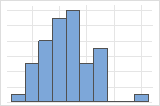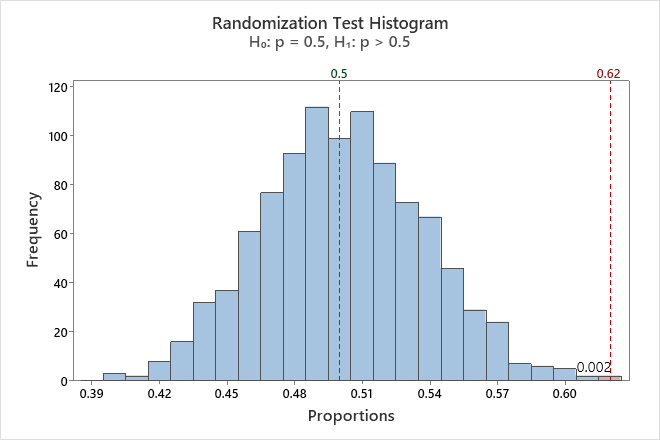# All statistics and graphs for Randomization test for 1-sample proportion

Find definitions and interpretation guidance for every statistic and graph that is provided with randomization test for 1-sample proportion.

## Histogram

A histogram divides sample values into many intervals and represents the frequency of data values in each interval with a bar.

### Interpretation

Use the histogram to examine the shape of your bootstrap distribution. The bootstrap distribution is the distribution of means from each resample. The bootstrap distribution should appear to be normal. If the bootstrap distribution is non-normal, you cannot trust the results.The distribution is usually easier to determine with more resamples. For example, in these data, the distribution is ambiguous for 50 resamples. With 1000 resamples, the shape looks approximately normal.

The histogram visually shows the results of the hypothesis test. Minitab adjusts the data so that the center of the resamples is the same as the hypothesized mean. For a one-sided test, a reference line is drawn at the mean of the original sample. For a two-sided test, a reference line is drawn at the mean of the original sample and at the same distance on the opposite side of the hypothesized mean. The p-value is the proportion of sample means that are more extreme than the values at the reference lines. In other words, the p-value is the proportion of sample means that are as extreme as your original sample when you assume that the null hypothesis is true. These means are colored red on the histogram.In this histogram, the bootstrap distribution appears to be normal. The p-value of 0.002 indicates that 0.2% of the sample proportions are greater than the proportion of the original sample.

## Bar chart

The bar chart shows the proportion of occurrences for each category.

###### Note

Minitab displays a bar chart when you take only one resample. Minitab displays both the original data and the resample data.

### Interpretation

Use the bar chart to compare the original sample and the randomization sample. The randomization sample represents what a random sample would look like if the population mean was equal to the hypothesized value (the null hypothesis is true). The larger the difference between the centers of the two samples, the more evidence you would expect against the null hypothesis.

## Proportion

The sample proportion equals the number of events divided by the sample size (N).

### Interpretation

Minitab displays two different proportion values, the proportion of the observed sample and the proportion of the bootstrap distribution (Average). The proportion of the observed sample is an estimate of the population proportion. The proportion of the bootstrap distribution is usually close to the hypothesized proportion. The larger the difference between these two values, the more evidence you would expect against the null hypothesis.

## Null hypothesis and alternative hypothesis

The null and alternative hypotheses are two mutually exclusive statements about a population. A hypothesis test uses sample data to determine whether to reject the null hypothesis.
Null hypothesis
The null hypothesis states that a population parameter (such as the mean, the standard deviation, and so on) is equal to a hypothesized value. The null hypothesis is often an initial claim that is based on previous analyses or specialized knowledge.
Alternative hypothesis
The alternative hypothesis states that a population parameter is smaller, greater, or different than the hypothesized value in the null hypothesis. The alternative hypothesis is what you might believe to be true or hope to prove true.

### Interpretation

In the output, the null and alternative hypotheses help you to verify that you entered the correct value for the hypothesized proportion.

## Number of Resamples

The number of resamples is the number of times Minitab takes a random sample with replacement from your original data set. Usually, a large number of resamples works best.

Minitab adjusts the data so that the center of the resamples is the same as the hypothesized proportion. The sample size for each resample is equal to the sample size of the original data set. The number of resamples equals the number of observations on the histogram.

## Average

The average is the sum of the proportions in the bootstrapping sample divided by the number of resamples. Minitab adjusts the data so that the center of the resamples is the same as the hypothesized proportion.

### Interpretation

Minitab displays two different proportion values, the proportion of the observed sample and the proportion of the bootstrap distribution (Average). The proportion of the observed sample is an estimate of the population proportion. The proportion of the bootstrap distribution is usually close to the hypothesized proportion. The larger the difference between these two values, the more evidence you would expect against the null hypothesis.

## P-Value

The p-value is the proportion of sample proportions that are as extreme as your original sample when you assume that the null hypothesis is true. A smaller p-value provides stronger evidence against the null hypothesis.

### Interpretation

Use the p-value to determine whether the population proportion is statistically different from the hypothesized proportion.

To determine whether the difference between the population proportion and the hypothesized proportion is statistically significant, compare the p-value to the significance level. Usually, a significance level (denoted as α or alpha) of 0.05 works well. A significance level of 0.05 indicates a 5% risk of concluding that a difference exists when there is no actual difference.
P-value ≤ α: The difference between the proportions is statistically significant (Reject H0)
If the p-value is less than or equal to the significance level, the decision is to reject the null hypothesis. You can conclude that the difference between the population proportion and the hypothesized proportion is statistically significant. To calculate a confidence interval and determine whether the difference is practically significant, use Bootstrapping for 1-sample function. For more information, go to Statistical and practical significance.
P-value > α: The difference between the proportions is not statistically significant (Fail to reject H0)
If the p-value is greater than the significance level, the decision is to fail to reject the null hypothesis. You do not have enough evidence to conclude that the difference between the population proportion and the hypothesized proportion is statistically significant.
By using this site you agree to the use of cookies for analytics and personalized content.  Read our policy